TRYING TO UNDERSTAND THE ANDERSON DARLING TEST

by P Barber (March 2010)

(Back)

Introduction

1. The Anderson Darling test (Anderson Darling 1952) has been found to be one of the most effective tests for discriminating between candidates for the fitting of a distribution to a data set. (Stephens 1974). However, the author has struggled to understand how and why this test works. This paper is an attempt to set out the authors search for an explanation of this powerful test.

2. The results of the test are not dependent upon the particular distribution being selected, which means that the test is not limited to a few particular distributions. (Engineering Statistics Handbook)

3. The function underlying the test appears to be of the form: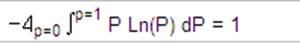4. Where P represents the cumulative distribution of idealised data points within the range zero to one. A plot of this function is shown below. The area under the graph is equal to one. Note that the curve below does not represent a statistical distribution, it might look like a frequency distribution curve, but it is a function, not a distribution.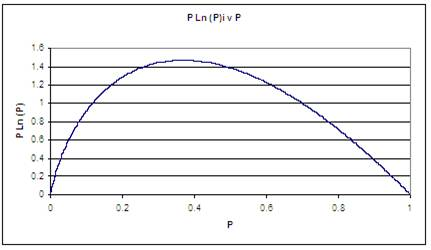5. As proof of the above equation, the website http:integrals.wolfram.com/index.jsp? Supplies the integral: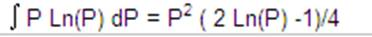6. Inserting the limits of P = 1 we have: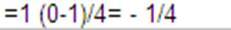7. And for P = 0 we have: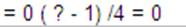8. We therefore have: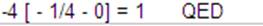9. When  calculating the Anderson Darling statistic in a spreadsheet, integration is carried out using N rows of data, rather than across the probability range of P = zero to 1. As a result the area under the graph is not equal to one, but to the value N = 1 x N10. The area under the graph show above is equal to N, the number of data elements, in the example shown N = 10,000. As the value of N is increased the value of P1, the smallest value of Pi, given by Pi = (2i  1)/2N where i = 1 is the elemental cumulative probability, tends towards zero. The equation for the ideal fit becomes: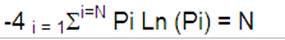11. The Anderson Darling Statistic measures the deviation away from this idealised situation, in which:

a) all the data elements are evenly spaced, such that their cumulative probabilities follow the form: Pi = (2i -1)/2N

b) the probabilities fitted to the data points Xi, defined as Pf  =  Pi, and

c) given that the probabilities are evenly spaced, Pi = P (N  i)

12. Hence the Anderson Darling elemental equation: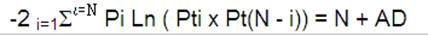13. Reduces to: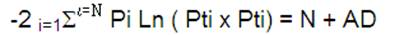14. Which when Pi = Pf = P(Nf) reduces to the equation shown above in paragraph 10.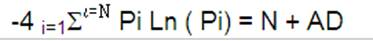Note that this equation could also be expressed in the form:THE ANDERSON DARLING EQUATION

15. The Anderson darling statistic measures the difference between the idealised form, as shown in the equation above, and that found in actual ranked data. Sorting the data points into ascending order is an important step and needs to be undertaken first.  The table below illustrates the process.

 a) b) c) d) e) f) g) h) i) Rank Data Pi Pf Ln(Pf) Ln(1-Pf) InvRank Ln(Pn-f) ADi 1 18,937 0.00005 0.0000001 -16.40826 0.000000 10000 -9.990734 0.002640 2 20,549 0.00015 0.0000086 -11.65808 -0.000009 9999 -9.851263 0.006453 3 21,946 0.00025 0.0000381 -10.17494 -0.000038 9998 -9.430134 0.009803 4 22,060 0.00035 0.0000418 -10.08291 -0.000042 9997 -8.662173 0.013122 5 22,183 0.00045 0.0000460 -9.98684 -0.000046 9996 -8.529218 0.016664 6 23,155 0.00055 0.0000888 -9.32937 -0.000089 9995 -8.008338 0.019071 7 23,559 0.00065 0.0001121 -9.09646 -0.000112 9994 -7.807460 0.021975 " " " " " " " " " " " " " " " " " " 9992 523,568 0.99915 0.9993558 -0.00064 -7.347528 9 -0.000228 0.001744 9993 524,362 0.99925 0.9993738 -0.00063 -7.375826 8 -0.000202 0.001656 9994 536,107 0.99935 0.9995933 -0.00041 -7.807460 7 -0.000112 0.001037 9995 541,350 0.99945 0.9996673 -0.00033 -8.008338 6 -0.000089 0.000843 9996 554,329 0.99955 0.9998024 -0.00020 -8.529218 5 -0.000046 0.000487 9997 557,505 0.99965 0.9998270 -0.00017 -8.662173 4 -0.000042 0.000429 9998 574,841 0.99975 0.9999197 -0.00008 -9.430134 3 -0.000038 0.000237 9999 583,662 0.99985 0.9999473 -0.00005 -9.851263 2 -0.000009 0.000123 10000 586,484 0.99995 0.9999542 -0.00005 -9.990734 1 0.000000 0.000092 Total ADi 10,000.205288 Less N = 10,000 10,000.000000 AD statistic 0.205288

16. The columns in the table above are defined as follows:

a)      Column a) shows a rank number for each element of data. This column is used to provide a row reference later in the process.

b)      Column b) shows the values of the dataset which is to be tested. This data must be ranked in ascending order.

c)      Column c) shows the value of Pi, which is a rank probability, calculated by the formula (2i  1)/2N, where i is the rank number and N the total number of values in the dataset. For example the value in the third row is calculated (2 x 3 -1)/2 x 10,000 = (5/20,000) = 0.00025

d)     Column d) shows the probability value Pf corresponding to the elemental value of the dataset. In the example the Excel function =BETADIST( data, Alpha, Beta, Minimum, Maximum) is used to calculate the value of Pf which would correspond to the data value under the distribution assumed. In this case the assumption that the data set corresponds to a Beta Distribution with parameters Alpha = 2.78692 Beta = 9.14524 Minimum = 18,581  Maximum = 760,332. Using these parameters in combination with a data value of 23,559 (column c) provides a Beta Cumulative Probability value of 0.0001121 which appears as the seventh item in column d).

e)      Column e) shows the natural logarithm of Pf. Referring to the seventh item Ln(0.0001121) = -9.09646

f)       Column f) shows the natural logarithm of  (1 - Pf) . Referring to the seventh item Ln( 1  0.0001121) =  - 0.00011

g)      Column g) shows a reverse ranking: the Rank sequence shown in column a) sorted descending. This column is used as a reference.

h)      Column h) uses a =VLOOKUP( ref, area, col, false) command, set to: = VLOOKUP( g, column a) to f) x N data elements, 6, false). The effect of this command is to select the value of Ln( 1  Pf) from the opposite end of the table. Referring to the seventh item in the table. The Inverse Rank appearing in column g) is equal to 9994, so the value of Ln(1 Pf) corresponding to the 9994th element in the table, of -7.807460 is selected.

i)        The elemental value of ADi is calculated in column i). The equation = -2 Pi [Ln(Pf) + Ln(Pn-f)] or referring to the columns in the table above this becomes  = - 2 x(c) x [(e) +( h)] duly the seventh item = -2 x 0.00065 x [ - 9.096460 + - 7.807460] = 0.021975

The value of the Anderson Darling statistic is then found by summing the elemental values in column i) and subtracting the value of N, the number of data elements. The sum of the elements = 10,000.205288 from which is subtracted N = 10,000 to provide a statistic of AD = 0.205288

17. The Anderson Darling statistic is affected by the value of N (the number of data elements) as shown in the graph below (authors tests based on Pi = Pf). The fitted equation shown can be rewritten as AD = 0.6714 Exp( -0.8809 Ln(N)), giving for N = 200: AD = 0.6714 Exp (-0.8809 Ln (200)) =  0.0063.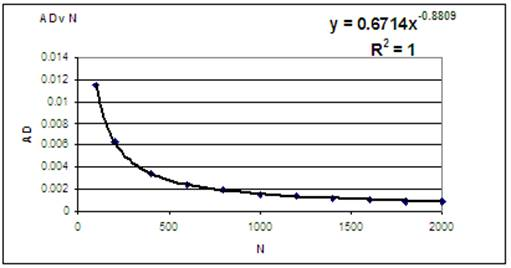18. Wikipedia (April 2010) suggests an adjustment to the value of AD in respect of the size of the dataset N and provides a formula for use with a normal distribution, where AD* = AD(1 + (4/N)  (25/N2)) and recommends that if AD* exceeds 0.751 then the hypothises of normality is rejected for a 5% level test; 0.632 for a 10% level 0.870 for a 2.5% level and 1.029 for a 1% level.

19. Annis (2009) and Romeu (2003-5) suggests an adjustment for the size of the dataset N, suitable for use with Normal and Lognormal distributions, where AD* = AD (1 + (0.75/N) + (2.25/N2)) with rejection of the hypothesis of normality if AD* is exceeds the values of: 0.631 at 10%, 0.752 at 5%, 0.873 at 2.5% and 1.035 at 1% levels of significance.

20. Annis (2009) and Romeu (2003-5) also suggests that for a Weibull and Gumbel distributions the value of AD* = AD(1 + 0.2/sqrt(N)) with rejection of the hypotheseis if the calculated value of AD* exceeds 0.637 at 10%, 0.757 at 5%, 0.877 at 2.5% and 1.038 at 1% levels of significance.

21. It is noted that the adjustments applied to the value of AD proposed in paragraphs 18, 19 and 20 would increase the value of AD, whereas the data derived in section 17 clearly shows that the value of AD decreases as the size of the dataset N, is increased. The data used to compile the graph in paragraph 17 was tested using both the Normal and Beta distributions and each distribution produced an identical result. It is not clear why it is believed (by Wikipedia, Annis and Rameu among others) that the type of the underlying distribution effects the formula for adjusting AD for the size of sample.

22. The three graphs below show the resolution of the statistic to determine the best-fit to the data set as the value of Alpha is varied from 6.0 to 10.0 The range of variation is reduced as the graphs move from left to right. These graphs are based on a dataset with N = 100.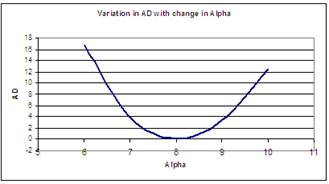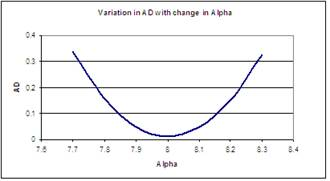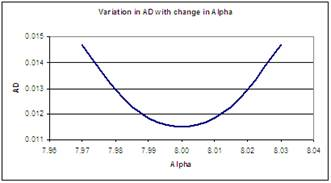23. The way in which the Anderson Darling function provides such a discriminating result is not easy to see (meaning that I do not understand it). Apart from the issue of ranking, there appear to be three elements in the equation making up the Anderson Darling (Engineering Statistic Handbook) calculation, namely:

a)      Pi where Pi = (2i-1)/N

b)      Ln (Pf)

c)      Ln (1  Pf)

The distribution of each of these elements is shown graphically below.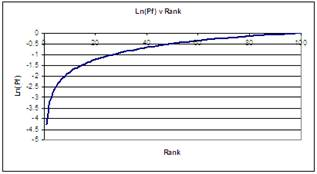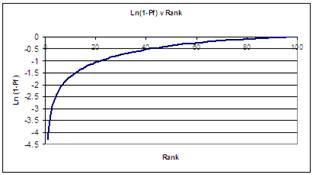24. As can be seen the form of Pi is linear, while the other two have a very similar form, indeed it would be difficult to distinguish any difference between these last two graphs visually (indeed the last two graphs are identical under the condition that Pf = Pi). A Graph showing the sum of the last two graphs is shown below.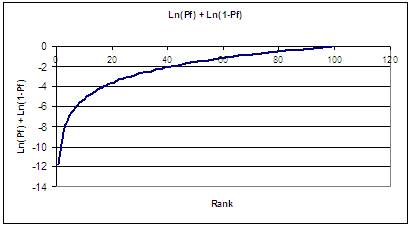25. The result of multiplying the sum of Ln(Pf) and Ln(1-Pf) by Pi and by -2 yeilds the elemental Anderson Darling statistic ADi and this is shown graphically below. The area under this graph is equal to (AD  N)26. The two graphs below illustrate the difference between the values of ADi for a curve which matches the underlying dataset and test curves in which the value of Alpha are varied between 6.0 and 9.0 The first graph shows the elemental differences, while the second graph shows the cumulative differences for the series of tests. The underlying dataset was created from a Beta Distribution with parameters: Alpha = 8  Beta = 10  Min = 20  Max = 5027. In the graphs above the value of Alpha takes on the values of 6, 7, 9 and 10. While the first graph illustrates that the distortion can occur in different forms and in different areas of the spectrum. It should be noted that is some areas of the spectrum (Alpha =6 and Alpha = 7, denoted by diff 6 and diff 7) the distortion is initially negative before going positive. The final result however, shown in the second graph is that the result is always positive, resulting in an increase in the value of the Anderson Darling statistic as the quality of the fitted distribution becomes more remote.

INDEPENDENT OF DISTRIBUTION BEING TESTED

28. An important feature of the Anderson Darling test is that it is independent of the distribution being tested. If  the Cumulative Probabilities (given by Pi = (2i  1) / 2N  Where i = the rank order and N = Total number of elements in the dataset) associated with a Ranked sample drawn at random are plotted against the ranked values (Xi), then the result is the familiar Cumulative Probability Distribution, or S-Curve.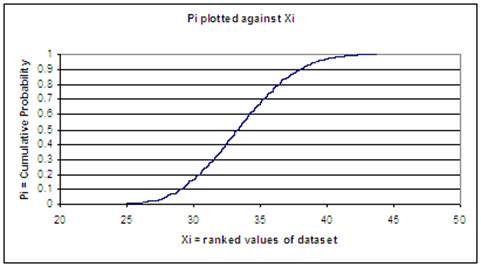29. In the Anderson Darling Test the ranked values of the dataset (Xi) are used to determine a probability Pf. The cumulative probability Pf represents the cumulative probability that the particular sampled value of the dataset Xi that would be expected if the sample had been drawn from a population with the distribution that had been assumed. For example, In the segment of data illustrated in the table below the ninth element of data has a value of Xi = 25.03353. If a Beta distribution with parameters Alpha = 8, Beta = 10, Minimum = 20 and Maximum = 50 has been assumed, then using the Excel Beta function it can be determined that the expected cumulative probability is Pf = 0.003622.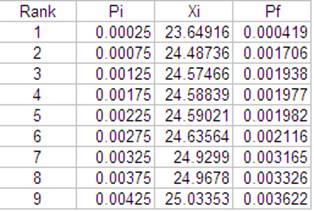30. If the values of Pf are plotted against Pi then if the assumed distribution fits the dataset, then the result will be a straight line.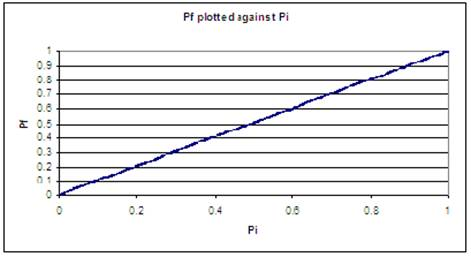31. The graph below assumes that the underlying distribution had parameters Alpha = 12, Beta = 10, Minimum = 20 and maximum = 50. The non-linear form of the graph clearly illustrates that these assumed parameters provide a very poor fit of the sampled data.32. The Anderson Darling test makes use of the linear property illustrated in paragraph 25 above, by utilising not only the value of Pf, but also the value of (1- Pf) from the opposite end of the distribution. It is the differences, or unevenness, in the cumulative probabilities, created by the assumed distribution which forms the basis of the test. As such the results of the test are independent of the form of the underlying sample distribution.

SIGNIFICANCE

33. The two segments of data in the table below compare the values produced by Crystal Ball when using the same sequence of random numbers. In the first segment a Beta Distribution, with parameters Alpha = 8, Beta = 10, Minimum = 20 and Maximum 50,  has been used to produce the values of Xi and to determine the values of Pf. In the second table a Normal Distribution, with parameters Mean = 35 and Standard Deviation = 5 has been used both to develop the values of Xi and compute the values of Pf. As can be seen the values of Pf in each table segment are the same. In fact if the Anderson Darling statistic is calculated for each table then it is found that a value of AD = 1.3527 is applicable to each; thereby demonstrating that the test is not only independent of the underlying distribution, but that the value of the statistic is dependent upon the sequence of random numbers selected.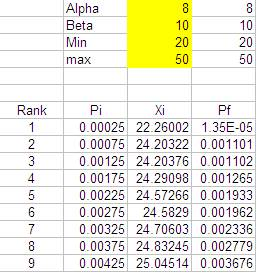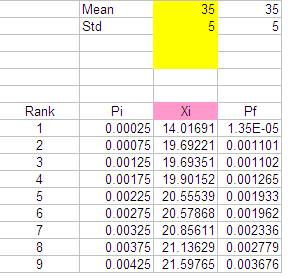34. The question being asked when considering significance is: If one were to extract a random sample from a larger dataset with a known distribution, and one were to then test this random sample against the known distribution, what would be the range of Anderson Darling statistic that might be expected? The graph below sets out the results of 200 trails (100 using the Beta Distribution and 100 using the Normal distribution as defined above) and plots the range of AD statistic that was encountered.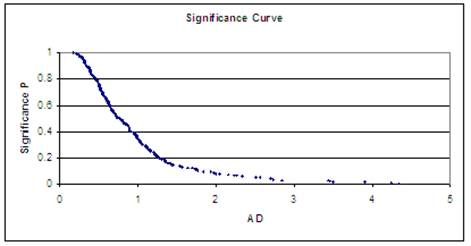35. A trendline has been added to this data in the graph below. Although this trendline is crude, it could provide a rough estimation of the significance level encountered.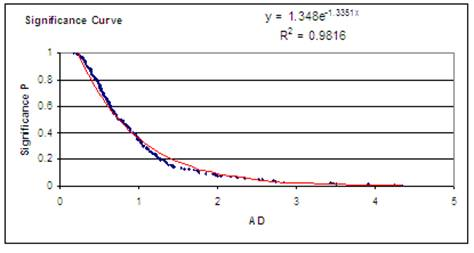36. While the graph above provides the basis for significance test, it should be noted that the individual values are a function of:

a) the sample size, and

b) the sample values selected from the general population and placed in the dataset.

37. When using Monte Carlo simulation the values selected are a function of the random numbers generated. Once again it should be noted that the form of the underlying distribution does not affect the value of the AD statistic, as demonstrated in the tables in paragraph 33 above. The significance graphs illustrated in paragraph 34 and 35 were developed using Oracle Crystal Ball, using the sampling method Mote Carlo (more random) and a sample size on N = 2000, while the graphs below show a significance curve developed using the Latin Hypercube method. The Latin Hypercube method provides a more even distribution and this is reflected in the lower AD values shown in the graph. The fitted trend line is based on a power series and gives a slightly better fit than a trend based on an exponentially factor. The difference in the levels of AD shown in the graph above and below is offered as proof that the shape of the significance curve is influenced significantly by the sampling method employed.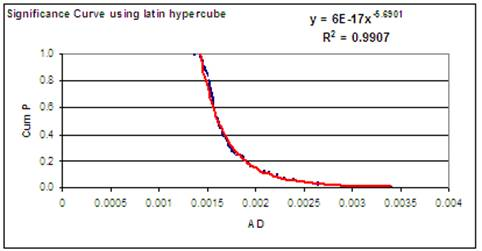38. As referred to in paragraph 20. both Annis (2009) and Romeu (2003-5) have suggested that different significance curves should be applied to different distributions. This assertion is disputed by the author, who believes that the results of the test are independent of the underlying distribution, but are dependent upon the random sequence used to select the data sample. As proof to this assertion the distributions shown below were tested using Oracle Crystal Ball employing a Latin Hypercube selection with a fixed starting seed of 999 and using a sample size of N = 2000. The Same result of AD =  0.002662093 was found to apply in each case, thereby verifying that the shape of the significance curve cannot be affected by the particular underlying distribution, but is affected by the random selection sequence. Note that the Maximum Extreme Distribution is the same as the Gumbel Distribution.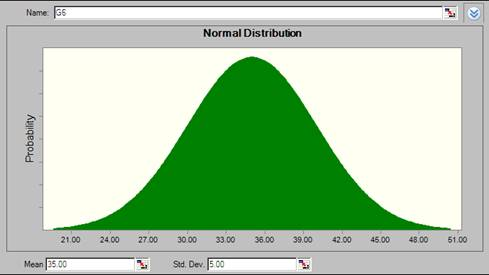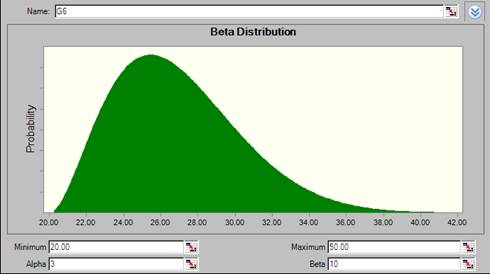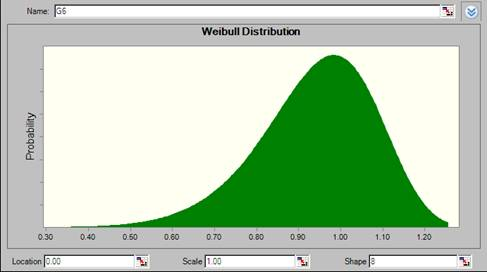CONCLUSION

39. It is concluded that the Anderson Darling Test provides a powerful method of assessing the goodness of fit of a distribution to a data sample, and the results of the test is independent of the underlying or assumed distribution. Significance levels are not affected by the distribution, but are affected by the size of the data sample and the method employed to produce the random dataset.

REFERENCES

Anderson, T. W.; Darling, D. A. (1952). Asymptotic theory of certain goodness-of-fit criteria based on stochastic processes. Annals of Mathematical Statistics 23: 193-212.

Annis C., ref: http://www.statisticalengineering.com/goodness.htm

Engineering Statistics Handbook. Ref http://itl.nist.gov/div898/handbook/eda/section3/eda35e.htm

Romeu J. L. Anderson-Darling: A Goodness of Fit Test for Small Samples Assumptions, START Selected Topics in Assurance Related Technologies, Vol 10, Number 5 (2003-5), ref: http://rac.alionscience.com

Stephens, M. A. (1974). EDF Statistics for Goodness of Fit and Some Comparisons. Journal of the American Statistical Association 69: 730-737

Wikipedia ( April 2010) ref: http://en.wikipedia.org/wiki/Anderson%E2%80%93Darling_test